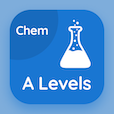Engineering Online Courses

Engineering Physics Quizzes

Engineering Physics Quiz PDF - Complete

# SI Derived Units Multiple Choice Questions p. 95

Study SI Derived Units multiple choice questions and answers, si derived units quiz answers PDF 95 to study Engineering Physics course online. Physics And Measurement MCQ trivia questions, SI Derived Units Multiple Choice Questions (MCQ) for online college degrees. "SI Derived Units MCQ" PDF eBook: gravitation near earth surface, introduction to thermodynamics, temperature and heat, relationship with constant angular acceleration, si derived units test prep for online engineering associate's degree programs.

"Linear acceleration is equals to" MCQ PDF: meter per second, square meter, meter per second per second, and radian per second for online engineering graduate colleges. Learn physics and measurement questions and answers to improve problem solving skills for best online colleges with financial aid.

## SI Derived Units Questions and Answers MCQs

MCQ: Linear acceleration is equals to

square meter
meter per second
meter per second per second

MCQ: Angular acceleration is denoted by

v
a
α
Ω

MCQ: SI unit of heat is

Btu
°F
J
°C

MCQ: Thermal energy is also known as

internal energy
external energy
optical energy
mechanical energy

MCQ: Communication satellite has gravitational acceleration of

9.83 m/s2
9.71 m/s2
8.70 m/s2
0.225 m/s2

### More Quizzes from Engineering Physics Course